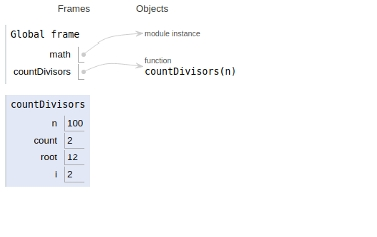# Python Program for Check if the count of divisors is even or odd

In this article, we will learn about the solution and approach to solve the given problem statement.

Problem statement −Given a number “n”, find its total number of divisors is even or odd.

In this approach, we will be finding all the divisors and checking that the count of divisors is even or odd.

The implementation is given below −

## Example

import math
def countDivisors(n) :
count = 0
# calculating all the divisors
root=int(math.sqrt(n))+2
for i in range(1, root) :
if (n % i == 0) :
# If divisors are equal,increment count by one Otherwise increment count by 2
if( n // i == i) :
count = count + 1
else :
count = count + 2

if (count % 2 == 0) : def countDivisors(n) :
count = 0
# calculating all the divisors
root=int(math.sqrt(n))+2
for i in range(1, root) :
if (n % i == 0) :
# If divisors are equal,increment count by one Otherwise increment count by 2
if( n // i == i) :
count = count + 1
else :
count = count + 2
if (count % 2 == 0) :
print("Even")
else :
print("Odd")
# Driver program to test above function */
print("The count of divisor: ")
countDivisors(100)
print("Even")
else :
print("Odd")
# Driver program to test above function */
print("The count of divisor: ")
countDivisors(100)

## Output

120
No

All the variables are declared in global scope as shown in the image below## Conclusion

In this article, we learned about the approach to Check if the count of divisors is even or odd of a given number.